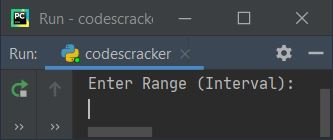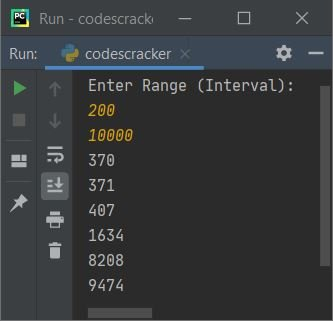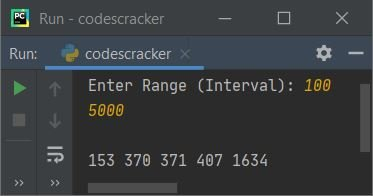# Python Program to Generate Armstrong Numbers

• Generate and Print Armstrong Numbers from 1 to 1000
• Generate and Print Armstrong Numbers in Given Range or Interval

Before creating these programs, let's remind about Armstrong numbers.

#### What are Armstrong Numbers ?

Any number that equals to sum of its own digits, where each digit raised to the power of number of digits. For example, 1634 is an Armstrong number. because:

```14 + 64 + 34 + 44
= 1 + 1296 + 81 + 256
= 1297 + 337
= 1634```

Note - Since 1634 is a 4-digit number, therefore each of its digit are raised to the power of 4

## Generate and Print Armstrong Numbers from 1 to 1000

To generate Armstrong numbers in python, you have to ask from user to enter the interval (starting and ending number), then generate and print Armstrong numbers between given range like shown in the program given below.

The question is, write a Python program to generate Armstrong numbers from 1 to 1000. Here is its answer:

```print("Armstrong Numbers Between 1 and 1000:")
first = 1
last = 1000
while first<=last:
res = 0
temp = first
noOfDigit = 0
while temp>0:
temp = int(temp/10)
noOfDigit = noOfDigit + 1
num = first
while num>0:
rem = num%10
pow = 1
i = 0
while i<noOfDigit:
pow = pow*rem
i = i+1
res = res+pow
num = int(num/10)
if res == first:
print(res)
first = first+1```

The snapshot given below shows the sample output produced by this Python program:The dry run of above program goes like:

• Initial values, first = 1, last = 1000
• Now the condition (of while loop) first<=last or 1<=1000 evaluates to be true, therefore program flow goes inside the loop or inside the block of while loop
• And 0 gets initialized to res, then first or 1 gets initialized to temp, and 0 gets initialized to noOfDigit
• Now the condition (of inner while loop) temp>0 or 1>0 evaluates to be true, therefore program flow goes inside this loop's block
• And int(temp/10) or int(1/10) or 0 gets initialized to temp. Then noOfDigit+1 or 0+1 or 1 gets initialized to noOfDigit
• first or 1 gets initialized to num and the condition num>0 or 1>0 evaluates to be true, therefore program flow goes inside this loop's block
• num%10 or 1%10 or 1 gets initialized to rem, 1 gets initialized to pow and 0 gets initialized to i
• The condition i<noOfDigit or 0<1 evaluates to be true, therefore program flow goes inside this loop's block and evaluates all its statement
• That is, pow*rem or 1*1 or 1 gets initialized to pow, i+1 or 0+1 or 1 gets initialized to i
• res+pow or 0+1 or 1 gets initialized to res, and int(num/10) or int(1/10) or 0 gets initialized to num
• Now the condition of if, that is res == first or 0 == 1 evaluates to be false, therefore program flow does not goes inside it's body. This condition is applied, to check and print Armstrong number.
• Then first+1 or 1+1 or 2 gets initialized to first
• Now program flow again evaluates the condition of second inner while loop
• That is, the condition num>0 or 0>0 evaluates to be false, therefore program flow does not goes inside this loop's block. Rather it goes and evaluates the condition of outermost (first) while loop
• That is, the condition first<=last or 2<=1000 evaluates to be true again therefore program flow again goes inside the loop.
• This process continues until the condition evaluates to be false

## Generate Armstrong Numbers in Given Range

This program receives interval (range) from user to generate and print Armstrong numbers between given range.

```print("Enter Range (Interval): ")
first = int(input())
last = int(input())

if first>last:
temp = first
first = last
last = temp

while first<=last:
res = 0
temp = first
noOfDigit = 0
while temp>0:
temp = int(temp/10)
noOfDigit = noOfDigit + 1
num = first
while num>0:
rem = num%10
pow = 1
i = 0
while i<noOfDigit:
pow = pow*rem
i = i+1
res = res+pow
num = int(num/10)
if res == first:
print(res)
first = first+1```

Here is its sample run:Now supply the input say 200 and 10000 as range or interval to find and print all Armstrong numbers between this range like shown in the snapshot given below:#### Modified Version of Previous Program

This is the modified version of previous program, uses try-except to handle with invalid inputs. The str() converts any type of value to a string type. The len() used in this program to find length of string (indirectly used to calculate number of digits):

```print("Enter Range (Interval): ", end="")
try:
first = int(input())
try:
last = int(input())
if first>last:
temp = first
first = last
last = temp
print()
while first<=last:
res = 0
noOfDigit = len(str(first))
num = first
while num>0:
rem = num%10
res = res + (rem ** noOfDigit)
num = int(num/10)
if res == first:
print(res, end=" ")
first = first+1
print()
except ValueError:
print("\nInvalid Input!")
except ValueError:
print("\nInvalid Input!")```

Here is its sample run with range input as 100 and 5000:#### Same Program in Other Languages

Python Online Test

« Previous Program Next Program »